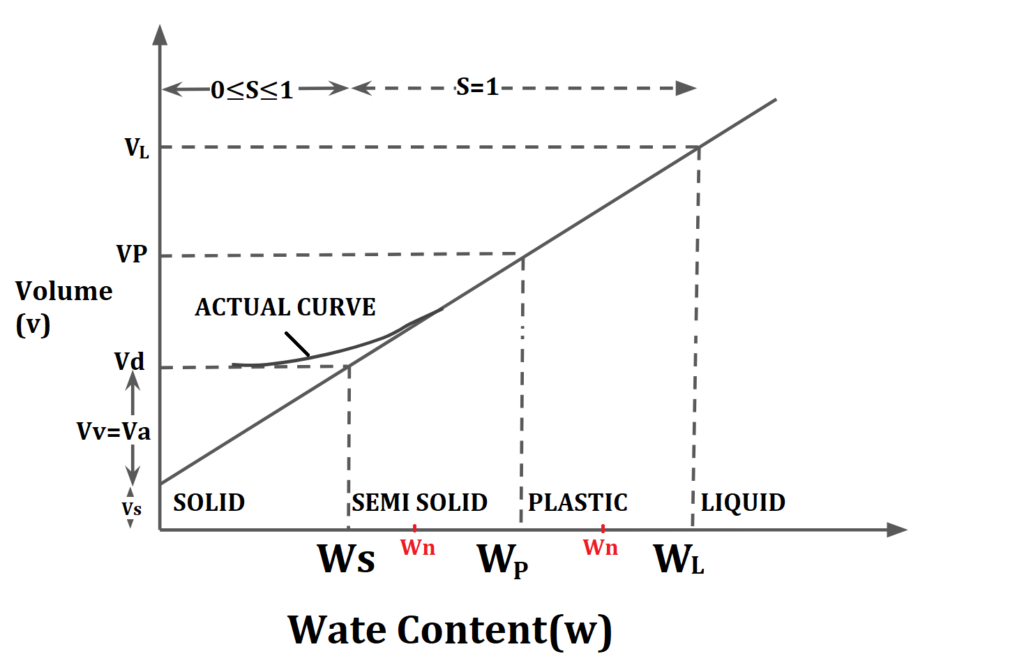# Consistency Index, Liquidity Index & Toughness Index | Properties of Soil | Soil Mechanics

Contents

## Consistency Index (Ic) /Relative consistency

In situ behavior of saturated fined grained soil at its natural water content is represented by consistency Index.

It is defined as ratio of difference of liquid limit and natural water content to the plasticity index.

$$I_c=\frac{w_L-w_n}{I_p}$$.

$$I_c=\frac{w_L-w_n}{w_L-w_p}$$.

Where

$$w_L$$= water content at Liquid Limit.

$$w_p$$= water content at Plastic Limit.

$$w_n$$= natural water content.## Liquidity Index (IL) /Water Plasticity Ratio

In situ behaviour of saturated fined grained soil at its natural water content is represented by consistency Index.

It is defined as ratio of difference of natural water content and plastic limit to the plasticity index.

$$I_L=\frac{w_n-w_p}{I_p}$$.

$$I_L=\frac{w_n-w_p}{w_L-w_p}$$.

Where

$$w_L$$= water content at Liquid Limit.

$$w_p$$= water content at Plastic Limit.

$$w_n$$= natural water content.NOTE-Sum of Consistency Index And Liquidity Index is always Unity.

$$I_L+I_C=\frac{w_n-w_p}{w_L-w_p}+\frac{w_L-w_p}{w_L-w_p}$$.

$$I_L+I_C=1$$.Note: Unconfined Compressive strength of soil is defined as load per unit area at which unconfined specimen of soil of standard dimension fail in simple compression test.

## Toughness Index (IT)

It represents the shear strength of soil at its plastic limit.

It is define as ratio of Plasticity Index &  Flow Index.

$$I_T=\frac{I_P}{I_p}$$.

$$I_T$$= Toughness Index.

$$I_P$$=Plasticity Index.

$$Ip = w_L-w_p$$.

$$I_F$$=Flow Index.

Generally :

0≤$$I_T$$≤3,

But if $$I_T$$<1 soil is considered to easily friable at plastic limit.

 Subject Soil Mechanics Unit Soil Formation & Properties of Soil Topic Consistency Index, Liquidity Index & Toughness Index Next Topic Sensitivity, Thixotropy & Activity Previous Topic Shrinkage Index, Shrinkage Ratio, Volumetric shrinkage, Linear Shrinkage, Plasticity Index

error: Content is protected !!
Scroll to Top